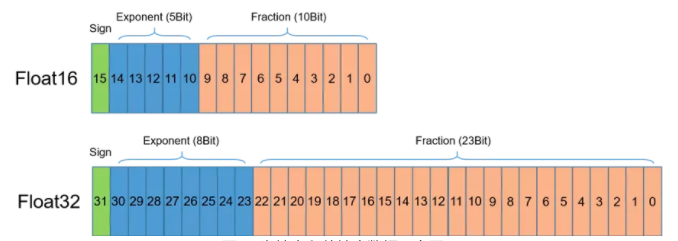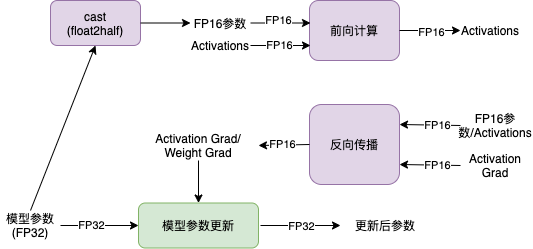# 自动混合精度¶

1. 减少对 GPU 显存的需求，或者在 GPU 显存保持不变的情况下，可以支持更大模型和更大的 batch size；

2. 降低显存读写的带宽压力；

3. 加速 GPU 数学运算速度 (需要 GPU 支持)；按照 NVIDA 数据，GPU 上`FP16`计算吞吐量是`FP32`的 2~8 倍

## 一、原理介绍¶• FP16 可降低一半的内存带宽和存储需求，这使得在相同的硬件条件下研究人员可使用更大更复杂的模型以及更大的 batch size 大小。

• FP16 可以充分利用英伟达 Volta 及 Turing 架构 GPU 提供的 Tensor Cores 技术。在相同的 GPU 硬件上，Tensor Cores 的 FP16 计算吞吐量是 FP32 的 8 倍。## 二、动态图操作实践¶

### 2.1 具体示例¶

```import time

# 开始时间
start_time = None

def start_timer():
# 获取开始时间
global start_time
start_time = time.time()

def end_timer_and_print(msg):
# 打印信息并输出训练时间
end_time = time.time()
print("\n" + msg)
print("共计耗时 = {:.3f} sec".format(end_time - start_time))
```

```import paddle

class SimpleNet(nn.Layer):

def __init__(self, input_size, output_size):
super().__init__()
self.linear1 = nn.Linear(input_size, output_size)
self.relu1 = nn.ReLU()
self.linear2 = nn.Linear(input_size, output_size)
self.relu2 = nn.ReLU()
self.linear3 = nn.Linear(input_size, output_size)

def forward(self, x):

x = self.linear1(x)
x = self.relu1(x)
x = self.linear2(x)
x = self.relu2(x)
x = self.linear3(x)

return x
```

```epochs = 5
input_size = 4096   # 设为较大的值
output_size = 4096  # 设为较大的值
batch_size = 512    # batch_size 为 8 的倍数
nums_batch = 50

train_data = [paddle.randn((batch_size, input_size)) for _ in range(nums_batch)]
labels = [paddle.randn((batch_size, output_size)) for _ in range(nums_batch)]

```

```model = SimpleNet(input_size, output_size)  # 定义模型

optimizer = paddle.optimizer.SGD(learning_rate=0.0001, parameters=model.parameters())  # 定义优化器

start_timer() # 获取训练开始时间

for epoch in range(epochs):
datas = zip(train_data, labels)
for i, (data, label) in enumerate(datas):

output = model(data)
loss = mse(output, label)

# 反向传播
loss.backward()

# 训练模型
optimizer.step()

print(loss)
end_timer_and_print("默认耗时:") # 获取结束时间并打印相关信息
```

```Tensor(shape=, dtype=float32, place=CUDAPlace(0), stop_gradient=False,
[1.25010288])

```

### 2.2 模型训练¶

1. 定义 GradScaler，用于缩放 loss 比例，避免浮点数下溢，即进行`loss scaling`

2. 使用 auto_cast 创建 AMP 上下文环境，该上下文中自动会确定每个 OP 的输入数据类型（FP16 或 FP32）。

3. 使用步骤 1 中定义的 GradScaler 完成 loss 的缩放，并用缩放后的 loss 进行反向传播，完成训练。

```model = SimpleNet(input_size, output_size)  # 定义模型

optimizer = paddle.optimizer.SGD(learning_rate=0.0001, parameters=model.parameters())  # 定义优化器

start_timer() # 获取训练开始时间

for epoch in range(epochs):
datas = zip(train_data, labels)
for i, (data, label) in enumerate(datas):

# Step2：创建 AMP 上下文环境，开启自动混合精度训练
output = model(data)
loss = mse(output, label)

# Step3：使用 Step1 中定义的 GradScaler 完成 loss 的缩放，用缩放后的 loss 进行反向传播
scaled = scaler.scale(loss)
scaled.backward()

# 训练模型
scaler.minimize(optimizer, scaled)

print(loss)
end_timer_and_print("使用 AMP 模式耗时:")
```

```Tensor(shape=, dtype=float32, place=CUDAPlace(0), stop_gradient=False,
[1.23644269])

```